# 渐开线绘图仪设计Design of Involute Drawing Plotter

• 全文下载: PDF(911KB)    PP.231-237   DOI: 10.12677/MET.2019.83029
• 下载量: 219  浏览量: 323

Aiming at the problem of involute drawing in engineering drawing class and related design drawing, an involute plotter is designed according to the generating principle of involute, and the design of the plotting tracks and key parts is studied. The plotter is mainly composed of gear and rack transmission mechanical system, centering device, and drawing device. By adjusting the centering device and the initial position of rack, the involute with different base circles and different starting positions can be drawn. The plotter has the advantages of simple structure, convenient carrying and high drawing precision. It is a powerful tool in the teaching of involute gear design and drawing.

1. 引言

2. 渐开线绘图仪的工作原理

2.1. 渐开线的产生原理

2.2. 渐开线绘图仪的结构原理1-定心装置；2-径向杆；3-齿轮；4-齿轮；5-齿条；6-齿轮；7-短轴；8-滚动论；9-手持盒

Figure 1. Axis profile diagram of plotter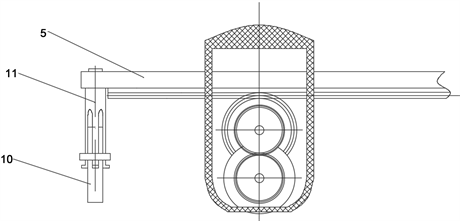5-齿条；10-粉笔；11-笔夹

Figure 2. Cross section diagram of plotter

3. 绘图轨迹计算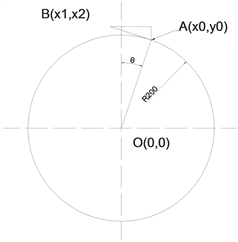Figure 3. Geometric relation diagram of plotter

$x0=R\cdot \mathrm{sin}\left(\theta \right)$ (1)

$y0=R\cdot \mathrm{cos}\left(\theta \right)$ (2)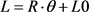(3)

$x1=d0\cdot \mathrm{sin}\left(\theta \right)-L\cdot \mathrm{cos}\left(\theta \right)$ (4)

$y1=d0\cdot \mathrm{cos}\left(\theta \right)+L\cdot \mathrm{sin}\left(\theta \right)$ (5)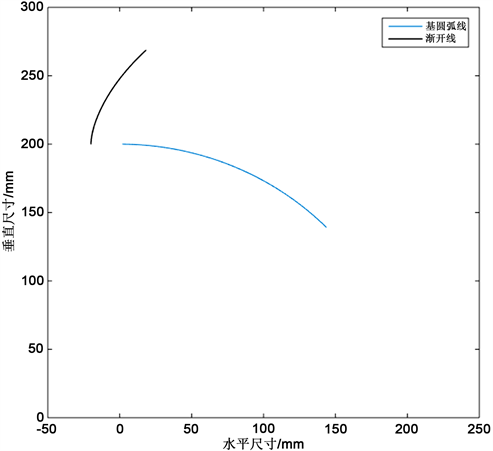Figure 4. Drawing trajectory calculation

4. 渐开线绘图仪的结构设计

4.1. 齿轮传动部分设计Table 1. Design parameters of gear transmission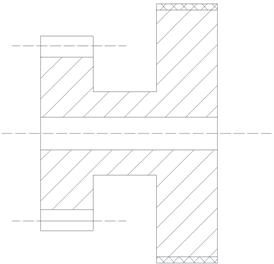Figure 5. Rolling wheel gear pair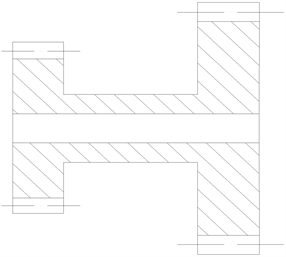Figure 6. Duplex gear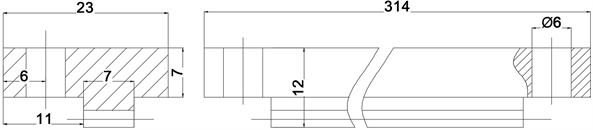Figure 7. Rack structure

4.2. 径向轴及圆心定位装置设计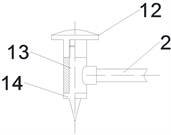2-径向杆；12-手柄；13-支架；14-定心针

Figure 8. Centering device structure

4.3. 笔架部分设计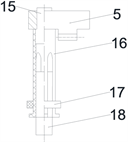5-齿条；15-阶梯轴；16-笔夹；17-紧固箍；18绘图笔

Figure 9. Drawing pen fixing device

5. 虚拟样机的制作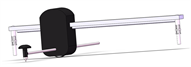(a)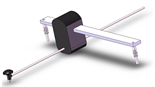(b)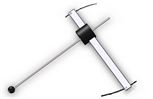(c)

Figure 10. Virtual prototype of involute plotter

6. 结论

1) 渐开线绘图仪实现工具绘制渐开线，改变现有手描方法绘制的缺陷，且绘图步骤简单、方便快捷、精确度高，是教学和学习的理想绘图工具。

2) 通过原理设计、轨迹计算和虚拟样机，对设计原理进行了验证，所设计绘图仪结构简单，使用方便，可绘制双向的渐开线。

  唐晓莲, 陈辽军. 一种弹簧片式渐开线绘图仪[P]. CN203282915U, CN201320341818.0.  魏军, 邱志敏. 基于ProE精确创建渐开线齿轮的基本原理及要求[J]. 现代设计与制造技术, 2007, 36(15): 35-37.  刘邢宇, 张学斌. 合肥工业大学渐开线绘图仪[P]. CN108454280A, CN201810194249.9.